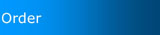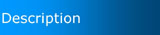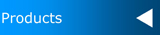ProductsFreefall7 is a 695 page ebook which is aimed at a average ability through to higher ability Year 7 student. It has 18 chapters which are: 01 Number 02 Shapes and Solids 03 Number Theory 04 Calculator 05 Measurement and Perimeter 06 Area 07 Time 08 Fractions 09 Decimals 10 Algebra 1 11 Angles 12 Integers 13 Algebra 2 14 Geometry 15 Graphs and Tables 16 Number Plane 17 Percentages 18 Volume Capacity and MassFreefall8 is a 971 page ebook which is aimed at a average ability through to higher ability Year 8 student. It has 16 chapters which are: 01 Calculator 02 Percentages 03 Pythagoras Theorem 04 The Circle 05 Area and Perimeter 06 Algebra 07 Volume and Capacity 08 Organising and Displaying Data 09 Equations and Inequations 10 Probability 11 Geometric Constructions 12 Rates and Ratio 13 Congruence and Similarity 14 Linear Relationships 15 Data Analysis 16 Surface AreaVelocity9 is a 747 page ebook which is aimed at a lower ability level, for Year 9 students. It has 7 chapters which are: 01 Earning Money 02 Area 03 Probability 04 Displaying Data 05 Indices 06 Numbers of any Magnitude 07 Taxation Velocity9 is smaller as it is anticipated that Year7 and Year 8 work won't be completed until the end of Term1 in Year 9. Subsequently the book is a three term book rather than a complete year, unlike all our other books.Velocity10 is a 1,090 page ebook which is aimed at a lower ability level, for Year 10 students. It has 12 chapters which are: 01 Data Analysis 02 Data Analysis Extension 03 Cartesian Plane 04 Investing Money 05 Investing Money Extension 06 Linear Relationships 07 Surface Area 08 Trigonometry 09 Trigonometry Extension 10 Non-Linear Relationships 11 Non-Linear Relationships Extension 12 Geometric Properties